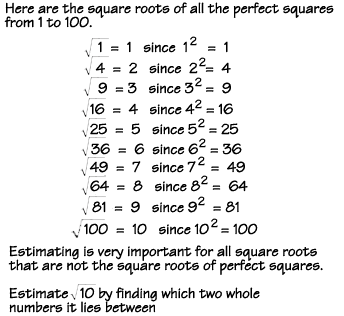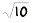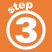Home    |    Teacher    |    Parents    |    Glossary    |    About UsExample: Calculate the square root of 10 () to 2 decimal places.

1. Find the two perfect square numbers it lies between.

Solution:
32 = 9 and 42 = 16, solies between 3 and 4.

2. Divide 10 by 3. 10/3 = 3.33 (you can round off your answer)

3. Average 3.33 and 3. (3.33 + 3)/2 = 3.1667

Repeat step 2: 10/3.1667 = 3.1579
Repeat step 3: Average 3.1579 and 3.1667. (3.1579 + 3.1667)/2 = 3.1623

Try the answer --> Is 3.1623 squared equal to 10? 3.1623 x 3.1623 = 10.0001

If this is accurate enough for you, you can stop! Otherwise, you can repeat steps 2 and 3.

 Homework Help | Pre-Algebra | NumbersEmail this page to a friendSearch·  Place value·  Decimal numbers·  Estimating and     rounding·  Adding / subtracting     decimals·  Multiplying decimals·  Dividing decimals·  Percent·  Exponents·  Square roots·  Signed integers·  Adding and     subtracting integers·  Multiplying and     dividing integers·  Properties of integersFirst Glance In Depth Examples WorkoutSquare roots